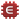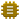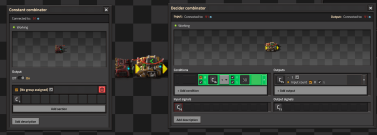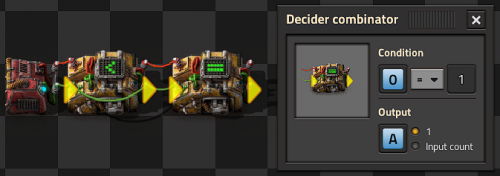# Tutorial:Combinator tutorial

This is an advanced tutorial. Beginners should refer to the Tutorial:Circuit network cookbook for examples and the Circuit network page for an overview over the circuit network. This tutorial assumes a basic understanding of circuits and covers more advanced topics like SR latches, memory cells and clocks.

## Introduction

Combinator logic is achieved by cross-connecting outputs to inputs in such a way to achieve the desired logic. While advanced logic requires a multitude of combinators, some very useful basic logic can be achieved using only a handful of combinators. Combinator logic works because Factorio only updates at 60 times per second, such that combinators analyze their logic in a step, and then sum and/or decide the resulting output values on the next step.

When logic values are computed by combinators, the outputs are not recognized by the circuit network until the following step. So when a decider combinator is used to detect a certain input condition, it's output value will not take effect on the circuit network until the next step. This behavior is important to remember and can result in sequencing errors and significant delays when multiple combinators are connected in series.

Circuit wires act like a wire bus in electronics; it carries information in the connected wires, meaning that if there are similar signals on a wire it will add them automatically. If the signal is different, it will be carried in that wire as well, but as a different signal.

When cross-connecting combinators, it is good practice to use the unused color to cross-connect, this will split the input and output networks and prevent unwanted inputs from accidentally connecting to a larger circuit network. Combinators will sum the red and green inputs prior to calculation, so either color can be used when wiring the output back to the input. In in most cases however, it is more useful to use the opposing color of the wire so that it will not interfere with the resulting output and input.

## Virtual signals

In addition to the standard item signals, Factorio's circuit network also includes a set of signals that do not represent any particular game item. Instead, these virtual signals serve as user-definable channels for the circuit network; they hold whatever meaning the user wants them to. There are currently 48 virtual signals that can be sent over the circuit network:

• The 36 alphanumeric characters (A-Z, 0-9)
• Nine colors: red, green, blue, yellow, magenta, cyan, white, grey, and black
• Three icons: a check mark, an informational letter 'i', and a small, white dot

### Logic signals

There are three additional virtual signals known as logic signals. Unlike other signals, they cannot be sent over the circuit network; instead, they apply additional logic to combinators that modify their behavior. Specifically, these logic symbols act as wildcards, which are special signals that represent zero or more arbitrary signals instead of representing a single discrete signal. Factorio's circuit network implements three types of wildcards.

#### Everything wildcardThe Everything wildcard is used with decider combinators. Its exact behavior depends on whether it is used as an input or an output:

• Input: Returns true if all input signals pass the condition, or if there are no input signals, otherwise it returns false.
• Output: Returns all non-zero input signals.

When used as an input, the everything wildcard can be thought of as a logical AND, or a universal quantifier. When used as an output, it acts as an 'echo' or 'dump' of input signals.

Note: Can be used as an output as long as the input is not an each wildcard.

#### Anything wildcardThe Anything wildcard is also used with decider combinators, but only as an input.

Given at least one input signal, it returns true if any input signal passes the condition. If no signal passes the condition, or there are no input signals, then it returns false. From this behavior, the anything wildcard can be thought of as a logical OR, or an existential quantifier.

#### Each wildcardThe Each wildcard is used with both decider combinators and arithmetic combinators, and behaves somewhat uniquely compared to the previous two. Generally speaking, it performs a combinator action on each signal individually, with the exact action depending on how it is used, and the type of combinator it is used in. It can be used as an input, and it can be used as an output, but only when it is used as input as well.

In a decider combinator, when used as an input, the each wildcard individually compares each input signal against the combinator condition, returning each signal that passes the condition. The manner that the each wildcard returns signals when used as input depends on whether or not it is also used as output:

• Input only: Sums each input signal that passed the condition, and depending on output settings returns either a count of the passed signals or a summation of their values as the desired output signal.
• Input and Output: Returns each signal that passed the condition, their values depending on the output settings.

In an arithmetic combinator, the designated arithmetic operation is applied individually to each input signal, and similar to the decider combinator, the signal that is returned depends on whether or not the each wildcard is used as output:

• Input only: The result of each operation on the input signals is summed and returned as the desired output signal.
• Input and Output: Each input signal is returned with the result of the specified operation applied to it.

The Each wildcard is therefore notably more complex than the other two wildcards, but offers a good deal of power in exchange for its complexity.

## Input insulator & gate

An arithmetic combinator set to (In: Each + 0, Out: Each) can be used to swap wire colors and as an insulator to prevent downstream logic from backfeeding into the circuit network's inputs.

A decider combinator set to (Out: Everything, Input-> Output) will also function as an insulator as long as the set logic condition is true. This can also selectively pass or 'gate' inputs only when desired. This could be used to sequentially poll remote train stations for their chest contents, and include only desired stations.

## Set/Reset latching switch

You want something to SET a trigger at some quantity, but then STAY on until that quantity hits some other value, the RESET value. You'll need one decider combinator and one arithmetic combinator. (Two decider combinators and a constant combinator can also be used for more complex multi-channel conditions.)

Setup the first decider combinator to the desired set conditional and to output a 1. Then connect the output to the input of an arithmetic combinator, and configure it to multiply by the bias value, which is the difference between the set and reset values, and wire the arithmetic output to the input of the decider. The arithmetic output channel must be set the same as the decider's input channel.
Whenever the set conditional is reached, the decider will output a '1', and the bias of the arithmetic combinator will be applied. This will 'hold' the output true until the value goes back below the reset point.

In this specific example, the pump runs when light oil reaches 20000, and turns off when it reaches 5000:

A similar backup steam power example with detailed configuration and explanation can be found here:
RS latch - single decider version

This solves the problem of loading logistics into chests, which tend to be unequal and is slower in the rate of loading logistics into the cargo of trains.

To setup the design, you require an Arithmetic Combinator, as well as Red and Green wires. Wire all the chests used, to the input of the Arithmetic Combinator. Then write (Logistics Item / -Amount of chests) and as the output as the Logistics Item in the Arithmetic Combinator, this will average the amount of items within the chests. Lastly, wire all the inserters used to the output of the Arithmetic Combinator and have the other color of the wire be wired to the adjacent chest. Have the inserters enabled when Logistics Item < 1.

A more visual representation as well as questions about the design can be found in a reddit post: MadZuri's smart loading train station.

Explanation of why this works: It compares the average amount of total items within the chests and the chest adjacent to the inserter so that it activates when the average number of items is higher than the amount within the chest. The reason for why the division denominator is negative is because if the items in the chests are 0, it basically makes it so that it adds 1 to the equation.

## Memory

How to store a constant value for later use, either for a basic counter or for more advanced logic. A decider combinator wired output tied to input and configured greater than zero (for positive values), input -> output will 'hold' a value, as long as all other inputs on the network are zero.

Any non-zero input condition will create a basic clock; incrementing the stored value by the sum of all connected input values every cycle. A single pulse of an input will cause a single increment by the pulsed value. Reset to zero occurs whenever the set condition is no longer met, or if a negative pulse equal to the input occurs.

## Basic clocksA basic clock. 30 ticks is the ceiling for Signal 1; which is continuously added.

Clocks are constructed by having the output of a combinator tied back to its own input, such that every cycle advances its own count. Either the arithmetic combinator or the decider combinator can be used.

An arithmetic combinator tied to itself is fun to watch and will happily run-away, but requires additional control logic to reset.

A self-resetting clock requires just a single decider combinator with output wired to input and configured with Less Than (<) and Input -> Output. When a constant combinator is then connected to the input, every cycle it will count up by the value of the Constant Combinator until the set conditional value is reached, then output a zero which will be summed with the constant combinator, and reset the process.

The clock sequence will not include zero, will begin at the value set by the constant combinator, and will include whatever value eventually causes the conditional to be false. An arithmetic combinator can modify the clock sequence but remember its outputs will occur one cycle later than the clock cycle values.

A clock that only counts once can be built using the following setup:

## Pulse generators

Connecting an additional (=) decider combinator to the output of a basic clock will create a pulse generator, and will pulse a single output every time the clock cycles through the set condition. Any output value can be used, either directly from the clock sequence (input->output), a 1, or some value on a separate logic channel on the circuit network, such as set by a constant combinator. or by the circuit network.• The value 1 can be written as any positive integer, so long as it is within the cap or ceiling of your timer.
• As an example from the above timer, this light will pulse every 1st tick after the timer reaches 30 ticks, making it pulse 1/30th of a second, as Factorio updates at 60 times per second.

## Counter

A counter is used to count the number of input events, and output the sum of that count. Any pulsing input into a decider combinator configured input -> output and wired between output and input will create a counter, but this input must be zero at all other times or else the combinator will run away like a clock. A pulse generator is normally used to accomplish this. Combining several gating decider isolators set with sequential conditionals, a clock, and a pulse generator to the input of a counter will allow remote polling and counting of each isolator's contents.

## Logic gates

Some logic gates will only function with boolean values, where the inputs are either 0 or 1. Other logic gates will work with other values as well, e.g. 2.

### Built In

The arithmetic combinator has built in OR, XOR and AND operators. These operate bitwise, i.e. they perform the operation on each bit in the number in a binary format and return the result.

An example for the bitwise AND operator:

Input 1 Input 2 Output
0 1 0
1 1 1
1 4 0
1 3 1
10 30 10
10 40 8

If your inputs only consist of boolean values (1 or 0) the arithmetic combinator's operators can be used as logic gates.

Truth Table:

Input Output
0 1
1 0

### Binary OR

Truth Table:

Input 1 Input 2 Output
0 0 0
0 1 1
1 0 1
1 1 1

*Notes: This works for any number of inputs, all sent as the signal "A". This works for boolean inputs as well as other values.

### Binary NOR

Truth Table:

Input 1 Input 2 Output
0 0 1
0 1 0
1 0 0
1 1 0

*Notes: This works for any number of inputs, all sent as the signal "A". This works for boolean inputs as well as other values.

### Binary XOR

Truth Table:

Input 1 Input 2 Output
0 0 0
0 1 1
1 0 1
1 1 0

*Note: This only works for boolean inputs.

### Binary AND

Truth Table:

Input 1 Input 2 Output
0 0 0
0 1 0
1 0 0
1 1 1

*Note:The arithmetic combinator example provided works for non-boolean values, and will return non-boolean values when given them as input, but only if both values are non 0.

*Note:The decider combinator example provided only works for boolean values. It can be modified to have any number of inputs by changing the "1" shown to 1 less than the number of inputs, or the "2" shown to the number of inputs, as demonstrated below for a Ternary AND.

### Ternary AND

Truth Table:

Input 1 Input 2 Input 3 Output
0 0 0 0
0 1 0 0
0 0 1 0
0 1 1 0
1 0 0 0
1 1 0 0
1 0 1 0
1 1 1 1

### Binary NAND

Truth Table:

Input 1 Input 2 Output
0 0 1
0 1 1
1 0 1
1 1 0

*Note:This only works for boolean input values. It can be modified to have any number of inputs by changing the "2" shown to the number of inputs.

### Binary XNOR

Truth Table:

Input 1 Input 2 Output
0 0 1
0 1 0
1 0 0
1 1 1

*Note:This only works for boolean input values.

## Memory cells

### Simple latch

When looping the combinator to itself, use a different color of wire from your main inputs or outputs.

Truth Table:

Output 1 Input 1 Input 2 Output 1 (t+1)
0 0 0 0
0 1 0 1
0 0 1 0
0 1 1 0
1 0 0 1
1 1 0 1 (2)
1 0 1 0
1 1 1 1 (2)

Output 1 is the green wire loop seen in the picture, it carries the value to latch.

Input 1 is Set, while Input 2 is Reset.

### Positive cell

Cell for storing a positive value, with reset support:

Connect the desired value as signal I on the right side to set the memory cell and connect a negative value as signal I to reset the cell.

• The output of the memory cell is 2 mutually exclusive signals.
• In case input signal I > 0 then signal I is passed to the other side.
• In case input signal I is interrupted, then signal M is passed instead as a memory of previous input value.
• When input signal I is interrupted, it takes 2 ticks to switch to memory signal M.
• In case input I signal lasts only one tick then memory cell starts to cycle between the 2 previous values, tick by tick. Indefinitely.
• Switching is seamless, e.g. there are no ticks with empty signal.

### Positives and negatives cell

This cell can store negatives or positives. Reset is done on a dedicated line. Additionally, a 1-tick burst is handled properly. Forum post.

• The output M (memory) is the last non-zero input I (Input).
• A non zero R (reset) signal sets the output to zero.
• 1-tick bursts of R or I are handled properly.
• Negatives are handled properly.

## Multiplier and Dictionaries/Arrays

• Multiplying two signals together is simple and requires only a single combinator, however multiplying a set of signals is more complicated.
• A proof is shown below for the equation and why it works.
• A dictionary is a system that allows a value on a specific signal to be accessed. For example, A can contain many signals (either from a constant combinator or memory cell) and B can contain 1 of a specific signal (such as blue signal). What remains is the blue-signal value from A. This is because all the other signals are multiplied by 0.
• Arrays are similar to dictionaries, but instead of using a signal as a key, we use a number. Constant combinators are placed mapping each signal to a unique number (such as 1 yellow belt, 2 red belt, 3 blue belt, 4 burner inserter, etc). Then, use a combinator of "each = index OUTPUT 1 of each" and plug that in as the input to a dictionary.
```   ((A+B)^2 - (A-B)^2)/4 = AB
(A+B)^2 - (A-B)^2 = 4AB
(A^2 + 2AB + B^2) - (A^2 - 2AB + B^2) = 4AB
4AB = 4AB
```